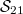﻿

### Classification of book spreads in PG(5, 2)

#### Abstract

We classify all line spreads$\mathcal{S}_{21}$ in(Error rendering LaTeX formula) of a special kind, namely those which are \emph{book spreads}. We show that up to isomorphism there are precisely nine different kinds of book spreads and describe the automorphism groups which stabilize them. Most of the main results are obtained in two independent ways, namely theoretically and by computer. \end

DOI Code: 10.1285/i15900932v33n2p43

Keywords: line spread; PG(5, 2); combinatorial design

Full Text: PDF